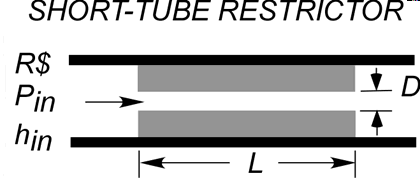Contents - index

Restrictor1_CLRestrictor1_CL provides an estimate of the mass flow rate through a short-tube restrictor with L/D ratios between 4 and 30 and a sharp-edged inlet for use in refrigeration cycles.  The mass flow rate is estimated from an empirical relation that has been derived based on data and other information in the following papers:

Aaron, D.A., and P.A. Domanski. 1990. Experimentation, analysis, and correlation of Refrigerant-22 flow through short tube restrictors.  ASHRAE Transactions 96(1):729-742.

Krakow, K. I., and Lin, S, 1988, Refrigerant flow through orifices, ASHRAE Transactions, Vol. 94, Part 1: 484-506.

Payne, W.V., and D.L. O'Neal. 1998. Mass flow characteristics of R-407 through short tube orifices. ASHRAE Transactions 104(1).

Payne, W.V., and D.L. O'Neal. 1999. Multiphase flow of refrigerant 410A through short tube orifices. ASHRAE Transactions 105(2).

Kim. Y. and O'Neal, D.L, 1994, Two-phase flow of R22 through short-tube orifices, ASHRAE Transactions 100(1)

Payne, W.V., and D.L. O'Neal. 1999. Multiphase flow of refrigerant 410A through short tube orifices. ASHRAE Transactions 105(2).

Inputs:

R\$:  name of the refrigerant

d:   inner diameter of the capillary tube (m, ft)

L:   length of the capillary tube (m, ft)

P_in:  pressure at the capillary tube inlet (Pa, kPa, MPa, bar, psia, atm)

h_in:  specific enthalpy at the restrictor inlet (J/kg, kJ/kg, Btu/lbm)

Output:

m_dot:  mass flow rate (kg/s, lbm/hr)

Example:

\$UnitSystem SI C kPa kJ mass

R\$='R22'

P_in=1.355 [MPa]*convert(MPa,kPa)

T_sat=temperature(R\$,P=P_in,x=0)

DELTAT_sc=5.6 [DELTAK]

h_in=enthalpy(R\$,T=T_sat-DELTAT_sc,P=P_in)

d=1.34 [mm]*convert(mm,m)

L=12.7 [mm]*convert(mm,m)

Call restrictor1_cl(R\$, d, L, P_in, h_in : m_dot)

m_dot_hr=m_dot*convert(kg/s,kg/hr)

{Solution:

m_dot=0.02896 [kg/s]

m_dot_hr=104.2 [kg/hr]

}